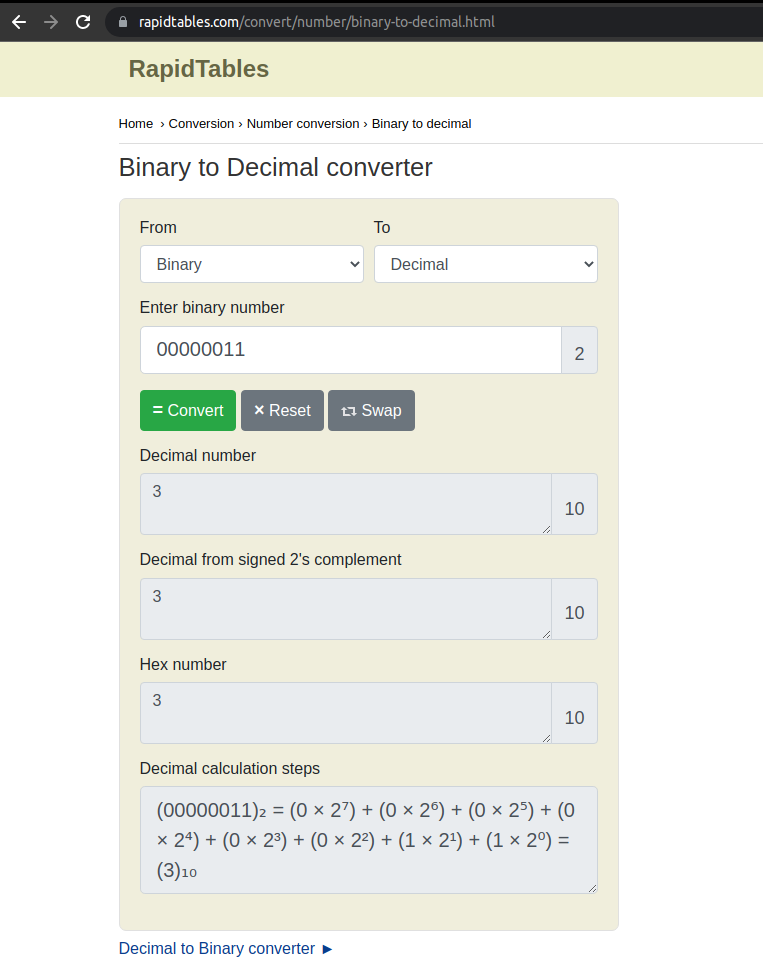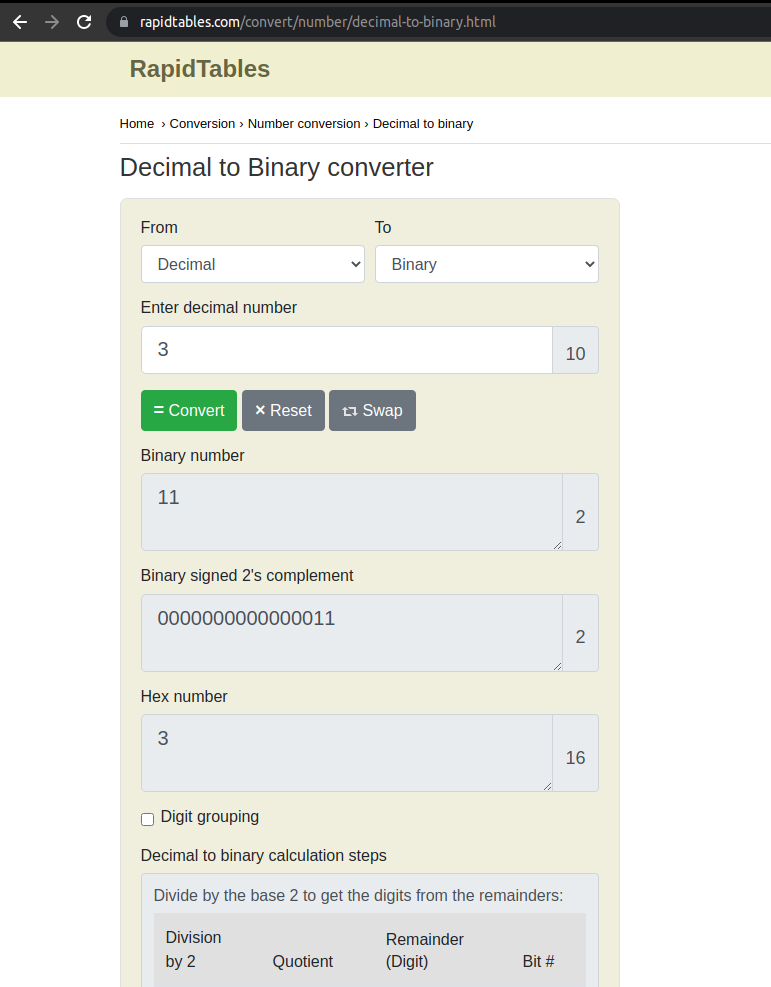Back-end Engineering Articles

I write and talk about backend stuff like Ruby, Ruby On Rails, Databases, Testing, Architecture / Infrastructure / System Design, Cloud, DevOps, Backgroud Jobs, and more...

@daniel_moralesp

2019-06-28

## Relationship Between Memory and Data Structures

So far, we've been talking about the introduction to data structures, and now we'll be talking about memory.

But we've to answer these questions first: why is memory so crucial at this point? Isn't it related to hardware? When we're programming and creating something, why is it important to consider the memory? Well, these are good questions, and actually, we can spend a lot of time explaining why.

The most straightforward answer is: because we need to take care of the resources involved in the project. When we talk about resources, we're talking about CPU, Processing, memory (and maybe other resources). That means that if we create programs with high resource consumption, the project owners can invest a lot of money in those physical resources.

So when we create algorithms or use data structures to create a solution to a problem, we've to monitor, test, and know the actual cost of our solutions to that problem and the best possible solution for it (the optimal one). So yes, we are talking a bit about hardware things here, but we'll see it with the eyes of a software developer, not from the hardware specialist's perspective.

Memory is an extensive concept, and we can spend a lot of time talking about the theoretical knowledge involved, so for now, we just want to do practical examples and have the right amount of knowledge from the software developer's perspective at a high level.

# Bits and Bytes

Bits and bytes are both data units, but what is the actual difference between them? One byte is equivalent to eight bits. A bit is considered to be the smallest unit of data measurement. The bit represents a logical state with one of two possible values. These values are most commonly defined as either "1" or "0"

```Bit == 1
Bytes == 00000001```

# Binary Numbers

Ok, so far, we're clear about the differences. This tends to confuse newbies in programming (I was also confused in my beginnings), but what we need to understand at this point is that bits and bytes are "nothing" without a conversion. Let me explain; if we have a variable called "my_number" and I'd assign the number 3 to it, now we have saved that value (3) in someplace in the memory to use it later while the program executes

So what we need to do is to convert that Integer 3 into a Binary Number (yes, we've to use a formula to do that, but that's not part of the post right now, and you can see more info here), and the result will be 00000011 (an 8-bit Integer).

LLet'scheck this web page, which helps us do this kind of conversion: https://www.rapidtables.com/convert/number/binary-to-decimal.html.And also, you can do the conversion by swapping the number to convert.In this last table, you'll see the output of the binary number, "11" because the zeros are omitted in the outcome.

I know this is hard to get at first, but as I mentioned before, the vital thing to remember now is that we have to convert the data types we've seen so far in Ruby, like Numbers, Strings, Arrays, and so on, into Binary Numbers. Actually, we don't do that, our machines do that for us, but this is one of the construction blocks to understand better why we'll need to optimize our solutions represented by algorithms.

Once we have the differences between bits and bytes more apparent, we can see more in detail memory with an example. Again, we'll be working with 4 different variables.

• ```var_a = 0
var_b = 1
var_c = 2
var_d = 3```

In the last section we learnt that we have to convert values (in this case assigned to a variable) into Binary Numbers (8-bit integer), so, the results we have once we do that are these ones:

• ```var_a = 0 ⇒ 00000000
var_b = 1 ⇒ 00000001
var_c = 2 ⇒ 00000010
var_d = 3 ⇒ 00000011```

# Memory slots & Memory addresses

Now we want to simulate how memory works internally. The first thing we have to take in mind is that memory is something finite, not infinite, and because of that, we have to take care of the optimization of our algorithms.

The second thing is that we have something called memory slots, and we can represent it as a table with its own interior spaces, and each internal space is represented by a memory address, in this case, the numbers we give (inside the table) to each area are from 0 to 15

Now let's simulate storing our 4 variables (converted previously into binary numbers) in these memory slots. Of course, we already have the binary digits (zeros and ones), storing them inside each memory slot.storing binary numbers

What we have now is

* we're simulating that memory addresses from 0 to 4 are complete (in use)
1. * then, we have a memory address associated with a binary number. For instance, address 5 is associated with 00000000
2. * and finally, from memory slot 9 to 15, we have free space for new binary numbers.

But what's happening from memory slot 5 to 8?
* memory address 5 is associated with 00000000
1. * then memory address 6 is associated with 00000001
2. * then we have a memory address number 8 associated with 00000011
3. * this last association means that binary number is (at the same time) associated with an integer. In this case, 00000011 is associated with integer number 3
4. * finally, we have that integer number 3 associated with the variable var_d

Things to note here:

• * All of these conversions are made by a computer at speed lightning
• * We didn't fill the first memory slots/addresses (intentionally) because we can say that they're already in use (storing other data)
• * We didn't fill the last memory slots/addresses (intentionally) because we can say that they're free for use (to store new data)

In computing, a memory address refers to a specific memory location used at various levels by software and hardware. Memory addresses are fixed-length digits conventionally displayed and manipulated as unsigned integers.

Such numerical semantic bases itself upon features of the CPU (such as the instruction pointer and incremental address registers) and upon the use of the memory like an array endorsed by various programming languages. More info about memory address here

# 32-bit integer

Now let's make a more realistic approach to memory usage. So far, we've been working with 8-bit integers (like 00000001 or 00000010)

Working with 32-bit integers are almost the same; we've just to add zeros in the memory slots until we reach the 32-bits number. Let me explain with an example where we have just this variable

• ```var_d = 3 ⇒ 00000011 (8-bit integer)
var_d = 3 ⇒ 00000000 00000000 00000000 00000011 (32-bit integer)```

The question now is how we save this data inside our memory slots? Each memory slot can just keep an 8-bit integer, so we need to use more memory slots to keep the number 3 converted to a 32-bit integer just like this.

As we can see, we're now storing just the value of 3 converted to a 32-bit integer, and we've assigned it to the addresses: 9, 10, 11, and 12. The first address contains the 8-bit integer, and the rest are filled with zeros. That's the way it works.

# 64-bit Integer

What happens if we want to save in a 64-bit integer? Let's do it

• ```var_d = 3 ⇒ 00000011 (8-bit integer)
var_d = 3 ⇒ 00000000 00000000 00000000 00000000 00000000 00000000 00000000 00000011 (64-bit integer)```

Now we've just to fill more memory slots like so
As we can see, we're now storing just the value of 3 converted to a 64-bit integer, and we've assigned it to the addresses: 9, 10, 11, 12, 13, 14, 15, and 16. The first address contains the 8-bit integer, and the rest are filled with zeros. That's the way it works.

Note: remember that all of this is a very high-level explanation but an easy way to understand the basics of memory.

# Pointers

So far, we have declared directly the values we need to store in each memory slot. However, sometimes we have just to retrieve information stored in other memory slots. So, we need to point to the address/address where the data is stored. It's simple like that. Let's see an example.

In this case, memory address #17 calls the value saved in memory address #9, which is 00000011, and with this, we're saving memory slots and re-using past data to retrieve even faster.

A pointer is an object in many programming languages that stores a memory address in computer science. This can be another value located in computer memory, or in some cases, a memory-mapped to the computer hardware.

A pointer references a location in memory, and obtaining the value stored at that location is known as dereferencing the pointer. The basic format and content of a pointer variable depend on the underlying computer architecture. More info here

# Run out of memory

As we've seen in this post, the memory slots are finite, which means you can run out of memory (probably you've lived it in the past). Memory allocation is something we've to understand for this very same reason. The question now is how to measure these things in our algorithms directly? The answer is given by the Big O Notation and is something we'll see in the next blog post.

I hope you learned a lot from this one.